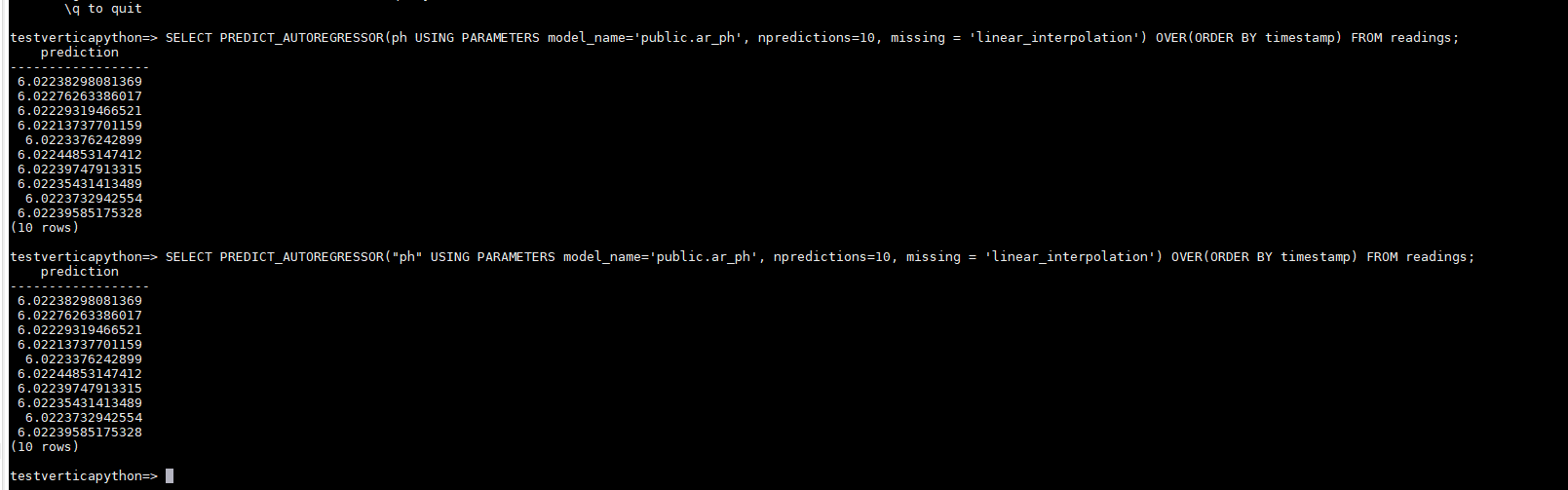The Vertica Forum recently got a makeover! Let us know what you think by filling out this short, anonymous survey.

# Error on PREDICT_AUTOREGRESSOR

edited November 2022

I followed the autoregressive model example but encountered an error when I tried to use predict_autoregressor to predict the future values.

https://www.vertica.com/docs/12.0.x/HTML/Content/Authoring/AnalyzingData/MachineLearning/TimeSeries/AutoregressorExample.htm

This is the SQL script I'm using
-- Create Auto Regressor Model for ph value
select AUTOREGRESSOR('public.ar_ph', 'AEROPREDICTIVEMAINT.readings', 'ph', 'timestamp' USING PARAMETERS p=3);

--Predict future value
SELECT PREDICT_AUTOREGRESSOR('ph' USING PARAMETERS model_name='public.ar_ph', npredictions=10, missing = 'linear_interpolation') OVER(ORDER BY timestamp) FROM AEROPREDICTIVEMAINT* .readings;

This is the error I received
[Code: 5861, SQL State: VP001] [Vertica]VJDBC ERROR: Error calling processPartition() in User Function predict_autoregressor at [src/Autoregression/PredictAR.cpp:281], error code: 0, message: Cannot linearly interpolate missing values when dataset starts with missing/invalid value(s). Either use a different 'missing' method or fix the dataset. Supported missing methods are 'error', 'zero', 'drop', and 'linear_interpolation'.

This is my data format
asset_id int
timestamp TIMESTAMP - format: YYYY-MM-DD HH:MI:SS
ph float
ec float

This is the sample data
1 1 2022-10-31 20:00:12 5.764902593 1077.394252
2 1 2022-10-31 20:00:27 6.820504555 1077.394252
3 1 2022-10-31 20:00:42 6.024084093 1076.323041
4 1 2022-10-31 20:00:57 6.020817099 1076.323041

I just tried to reproduce the issue locally and predict works when we use 'zero' option for missing parameter

(
asset_id int,
timestamp TIMESTAMP,
ph float,
ec float
);

```       4
```

(1 row)

```       4
```

(1 row)

## AUTOREGRESSOR

Finished. 8 elements accepted, 0 elements rejected.

(1 row)

============

# coefficients

parameter| value
---------+--------
alpha | 3.01265
phi_(t-1)| 0.74040
phi_(t-2)|-0.47930
phi_(t-3)| 0.23867

==================

# mean_squared_error

not evaluated

===============

# timeseries_name

ph

==============

timestamp

===========

# call_string

USING PARAMETERS p=3, missing=linear_interpolation, regularization='none', lambda=1, compute_mse=false);

===============

```   Name       | Value
```

------------------+--------
lag_order | 3
lambda | 1.00000
rejected_row_count| 0
accepted_row_count| 8

(1 row)

dbadmin=> SELECT PREDICT_AUTOREGRESSOR('ph' USING PARAMETERS model_name='public.ar_ph', npredictions=10, missing = 'zero') OVER(ORDER BY timestamp) FROM readings;

## prediction

3.01265249213884
5.24320767470682
5.45072628715033
5.25427583607328
5.54171723973476
5.89822460293606
5.97752349086881
5.93396319741793
5.94878916222428
5.99957076747547

• can you share me the output of the following?

SELECT GET_MODEL_SUMMARY(USING PARAMETERS model_name='public.ar_ph');

• Thanks a lot, I am able to use the predict_autoregressor by using the parameter "missing = 'zero'"

• @SruthiA
If I use predict_autoregressor with the parameter of "missing = 'zero' for EC value. The predicted value will be one digit but my ec value is in thousands digits. The sample data and data format are the same.

SELECT AUTOREGRESSOR('public.ar_ec', 'AEROPREDICTIVEMAINT.readings', 'ec', 'timestamp' USING PARAMETERS p=3);

SELECT PREDICT_AUTOREGRESSOR('ec' USING PARAMETERS model_name='public.ar_ec', npredictions=10, missing='zero' ) OVER(ORDER BY timestamp) FROM AEROPREDICTIVEMAINT.readings;

Prediction
0.5852650251472369
0.7919933213060548
1.0584737262246982
1.4056949353894361
1.681687556431865
1.978072541660872
2.283604735277106
2.5766226939424657
2.87468156635643
3.1732720688763987

• @yongwei : What happens when you use linear_interpolation for this table? Is it throwing same error?

Is is possible to open a support case and share around 1000 rows from table readings. It will help in understanding the data pattern and investigate on why autogressor model is predicting such low values.

• Do you have model generated for this table either in tensor flow or PMML? if so, could you please import that model into vertica and share model summary for the one which got generated using tensor flow or PMML and the one which was generated in vertica to review the coefficients etc

https://www.vertica.com/docs/12.0.x/HTML/Content/Authoring/SQLReferenceManual/Functions/MachineLearning/IMPORT_MODELS.htm

• @yongwei , you'll have to normalize your data.
I strongly encourage you to follow the "Data preparation" course in Vertica Academy
It's 100% free, and a hands-on lab is provided for one week:
You'll learn tons of stuff there (at least I did).

• I was trying other methods to see if it works, however due to less data present my cluster, drop method is not working. Could you please check if drop works for you?

testverticapython=> SELECT PREDICT_AUTOREGRESSOR('ph' USING PARAMETERS model_name='public.ar_ph', npredictions=10, missing = 'drop') OVER(ORDER BY timestamp) FROM readings;
ERROR 5861: Error calling processPartition() in User Function predict_autoregressor at [src/Autoregression/PredictAR.cpp:268], error code: 0, message: Insufficient number of data points provided. Must have at least p=3 valid elements in array.

• @yongwei The root cause of the issue is having single quotes for ph column. vertica is not able to recognize the column name when using single quotes. with regards to missing = zero, it was working because zero fills everything in with zeros.

Solution is to not use quotes or use double quotes

testverticapython=> SELECT PREDICT_AUTOREGRESSOR(ph USING PARAMETERS model_name='public.ar_ph', npredictions=10, missing = 'linear_interpolation') OVER(ORDER BY timestamp) FROM readings;

## prediction

6.02238298081369
6.02276263386017
6.02229319466521
6.02213737701159
6.0223376242899
6.02244853147412
6.02239747913315
6.02235431413489
6.0223732942554
6.02239585175328
(10 rows)

testverticapython=>

testverticapython=> SELECT PREDICT_AUTOREGRESSOR("ph" USING PARAMETERS model_name='public.ar_ph', npredictions=10, missing = 'linear_interpolation') OVER(ORDER BY timestamp) FROM readings;

## prediction

6.02238298081369
6.02276263386017
6.02229319466521
6.02213737701159
6.0223376242899
6.02244853147412
6.02239747913315
6.02235431413489
6.0223732942554
6.02239585175328
(10 rows)

testverticapython=>